Welcome to Wall Street Prep! Use code at checkout for 15% off.# Dividend Discount Model (DDM)

Guide to Understanding the Dividend Discount Model (DDM)## How Does the Dividend Discount Model (DDM) Work?

The dividend discount model (DDM) is a method used to estimate the intrinsic value of a stock based on the concept that the share price is worth the present value (PV) of the underlying issuer’s expected dividends.

Given the current stock price of the company, the appropriate discount rate (i.e. required rate of return) based on its risk-return profile, and the expected value of the next year’s dividend, the present value (PV) of all future dividends represents the implied intrinsic value.

Under the dividend discount model (DDM), the value per share of a company is equal to the sum of the present value of all expected dividends to be issued to shareholders.

Although a subjective determination, valid claims could be made that the free cash flow calculation is prone to manipulation through misleading adjustments.

Under the strictest criterion, the only real “cash flows” received by shareholders are dividend payments – hence, using dividend payments and the growth of said payments are the primary factors in the DDM approach.

## Dividend Discount Model Formula (DDM)

The formula to calculate the implied stock price under the dividend discount model (DDM) is as follows.

Intrinsic Value Per Share = D1 ÷ (ke g)

Where:

## What are the Two-Stage vs. Multi-Stage DDM Variations?

There are several variations of the dividend discount model (DDM) with the maturity and historical payout of dividends determining which appropriate variation should be used.

As a general rule, the more mature the company and the more predictable the dividend growth rate (i.e. an unchanged policy with a stable track record), the fewer stages the model will be comprised of.

But if dividend issuances have been fluctuating, the model must be broken into separate parts to account for the unstable growth.

## Multi-Stage DDM vs. Gordon Growth Model (GGM): What is the Difference?

Multi-stage dividend discount models tend to be more complicated than the simpler Gordon Growth Model, because, at the bare minimum, the model is broken into 2 separate parts:

1. Initial Growth Stage → Higher, Unsustainable Dividend Growth Rates
2. Constant Growth Stage → Lower, Sustainable Dividend Growth Rates

In effect, the estimated share price accounts for how companies adjust their dividend payout policies as they mature and reach the later stages of the forecast.

For instance, unlike the Gordon Growth Model – which assumes a fixed perpetual growth rate – the two-stage DDM variation assumes the company’s dividend growth rate will remain constant for some time.

At some point, the growth rate is then decreased as the growth assumption used in the first stage is unsustainable in the long term.

## What are the Different Types of Dividend Discount Model (DDM)?

• Zero Growth: The simplest variation of the dividend discount model assumes the growth rate of the dividend remains constant into perpetuity, and the share price is equal to the annualized dividend divided by the discount rate.
• Gordon Growth DDM: Frequently called the constant growth DDM, as implied by the name, the Gordon Growth variation assigns a perpetual dividend growth rate with no change throughout the entirety of the forecast.
• Two-Stage DDM: Considered a “multi-stage” DDM, the two-stage DDM determines the value of a company’s share price with the model split between an initial forecast period of increased dividend growth and then a period of stable dividend growth.
• Three-Stage DDM: An extension of the two-stage DDM, the three-stage variation consists of three stages, with the dividend growth rate declining over time.

## DDM vs. DCF Valuation: What is the Difference?

The dividend discount model (DDM) states that a company is worth the sum of the present value (PV) of all its future dividends, whereas the discounted cash flow model (DCF) states that a company is worth the sum of its discounted future free cash flows (FCFs).

While the DDM methodology is relied upon less by equity analysts and many nowadays view it as an outdated approach, there are several similarities between the DDM and DCF valuation methodologies.

 Discounted Cash Flow (DCF) Dividend Discount Model (DDM) The DDM forecasts a company’s future dividend payments based on specific dividend per share (DPS) and growth rate assumptions, which are discounted using the cost of equity. The DCF, on the other hand, projects a company’s future free cash flows (FCFs) based on discretionary operating assumptions such as profitability margins, revenue growth rate, free cash flow conversion ratio, and more. For calculating the terminal value, an equity value-based multiple (e.g. P/E) must be used if the exit multiple approach is used. And for the terminal value calculation, the exit multiple used can be either an equity value-based multiple or enterprise value-based multiple – depending on whether the DCF is on a levered or unlevered basis.

Upon completion, the DDM directly calculates the equity value (and implied share price) similar to levered DCFs, whereas unlevered DCFs calculate the enterprise value directly – and would require further adjustments to get to equity value.

## Cost of Equity in Dividend Discount Model (DDM)

The projected cash flows in a DDM – the dividends anticipated to be issued – must be discounted back to the date of the valuation to account for the “time value of money”.

The discount rate used must represent the required rate of return (i.e. the minimum hurdle rate) for the group of capital provider(s) who receive or have a claim to the cash flows being discounted.

With that said, the appropriate discount rate to use in the DDM is the cost of equity because dividends come out of a company’s retained earnings balance and only benefit the company’s equityholders.

On the income statement, if you imagine going down from “top-line” revenue to the “bottom-line” net income, payments to lenders in the form of interest expense affect the ending balance.

Net income is thus considered a post-debt, levered metric.

## What are the Shortcomings of Dividend Discount Model (DDM)?

Compared to its more widely used counterpart, the discounted cash flow model, the dividend discount model is used far less often in practice.

To some degree, all forward-looking valuations are flawed – with the DDM being no exception.

In particular, some of the drawbacks to the DDM method are:

• Sensitivity to Assumptions (e.g. Dividend Payout Amount, Dividend Payout Growth Rate, Cost of Equity)
• Reduced Accuracy for High-Growth Companies (i.e. Negative Denominator if Unprofitable, Growth Rate > Cost of Equity)
• Declining Volume of Corporate Dividends – Opting for Share Repurchases Instead
• Neglects Share Buybacks (i.e. Repurchases are Major Considerations for All Stakeholders and Outside Spectators in the Market)

The DDM is more suitable for large, mature companies with a consistent track record of paying out dividends. Even then, it can be very challenging to forecast out the growth rate of dividends paid.

In a perfect world where all corporate decisions were made by the book, dividend payout amounts and growth rates would be a direct reflection of the true financial health and expected performance of a company.

But the reality of the situation is that even poorly run companies could continue to issue large dividends, causing potential distortions in valuations.

The decision to issue large dividends could be attributable to:

1. Upper-Level Mismanagement: Management could be missing opportunities for re-investing into their core operations and instead focus on creating value for shareholders by issuing dividends.
2. Share Price Reduction Concern: Once implemented, companies rarely cut or end a previously announced dividend issuance program, as it serves as a negative signal to the market, which most investors interpret in the worst possible way.

## How is DDM Used to Value Commercial Banks?

Commercial banks are well-known for issuing relatively large dividend payouts consistently. The dividend discount model (DDM) is thereby frequently used in such instances.

The multi-stage DDM is most common for bank valuation models, which split up the forecast into three distinct stages:

1. Development Growth Stage: The forecasted dividend issuances are explicitly made and then discounted to the present using the cost of equity.
2. Maturity Growth Stage: The projected dividends are based on the assumption the company’s return on equity and cost of equity will converge (i.e. mature companies cannot sustain a return on equity much greater than their cost of equity into perpetuity).
3. Terminal Growth Stage (Perpetual): The final phase represents the present value of all future dividends once the company has reached maturity with a 1) perpetual dividend growth rate or 2) terminal equity value-based multiple being used.

## Dividend Discount Model Calculator (DDM)

We’ll now move on to a modeling exercise, which you can access by filling out the form below.Submitting...

## 1. Two-Stage Dividend Discount Model Assumptions

For our DDM modeling example exercise, the following assumptions will be used:

• Dividends Per Share (DPS) – Current Period: \$2.00
• Cost of Equity (Ke): 6.0%
• Dividend Growth Rate (g) – Stage 1: 5.0%
• Dividend Growth Rate (g) – Stage 2: 3.0%

To summarize, the company issued \$2.00 in dividends per share (DPS) as of Year 0, which will grow at a rate of 5% across the next five years (Stage 1) before slowing down to 3.0% in the perpetuity phase (Stage 2).

Regarding the company’s risk/return profile, our company’s cost of equity is 6.0% – the minimum return required by equity holders.

## 2. Two-Stage Dividend Discount Model Calculation Example

Once we have entered the model assumptions, we’ll create a table with the explicit present value (PV) of each dividend in Stage 1.

The formula for discounting each dividend payment consists of dividing the DPS by (1 + Cost of Equity) ^ Period Number.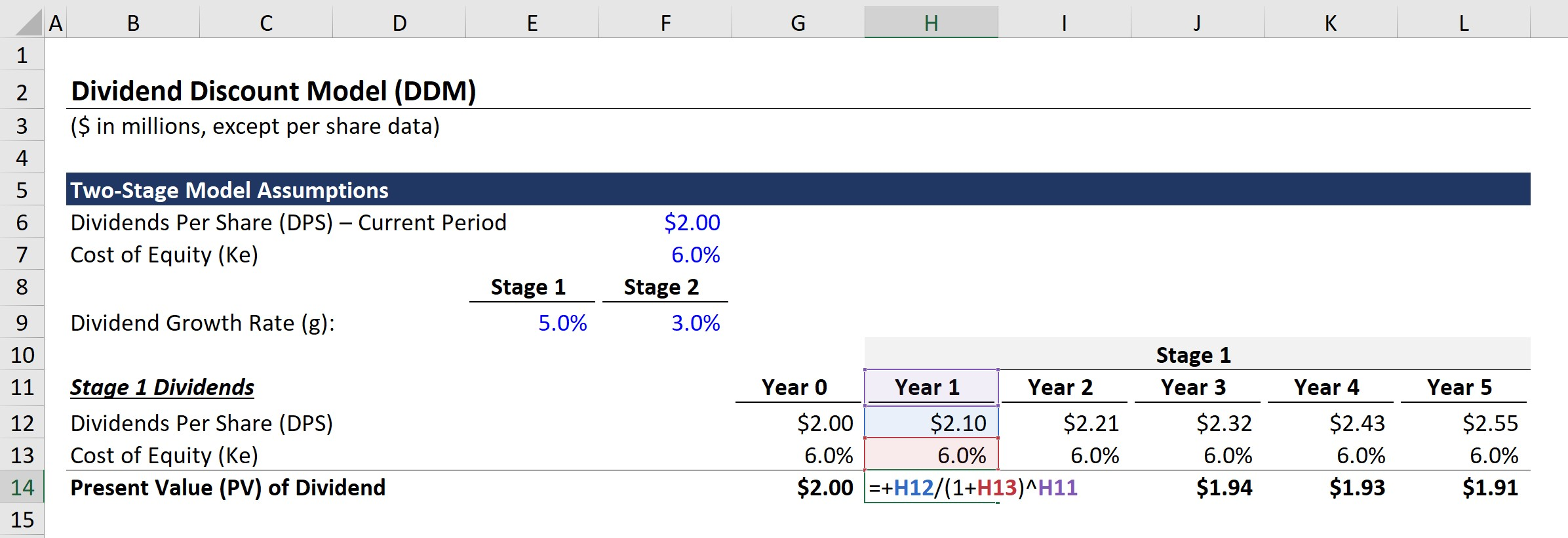After repeating the calculation for Year 1 to Year 5, we can add up each value to get \$9.72 as the PV of the Stage 1 dividends.

Next, we’ll move to Stage 2 dividends, which we’ll start by calculating the Year 6 dividend and entering the value into the constant growth perpetuity formula.

Upon multiplying the DPS of \$2.55 in Year 5 by (1 + 3%), we get \$2.63 as the DPS in Year 6. Then, we can divide the \$2.63 DPS by (6.0% – 3.0%) to arrive at \$87.64 for the terminal value in Stage 2.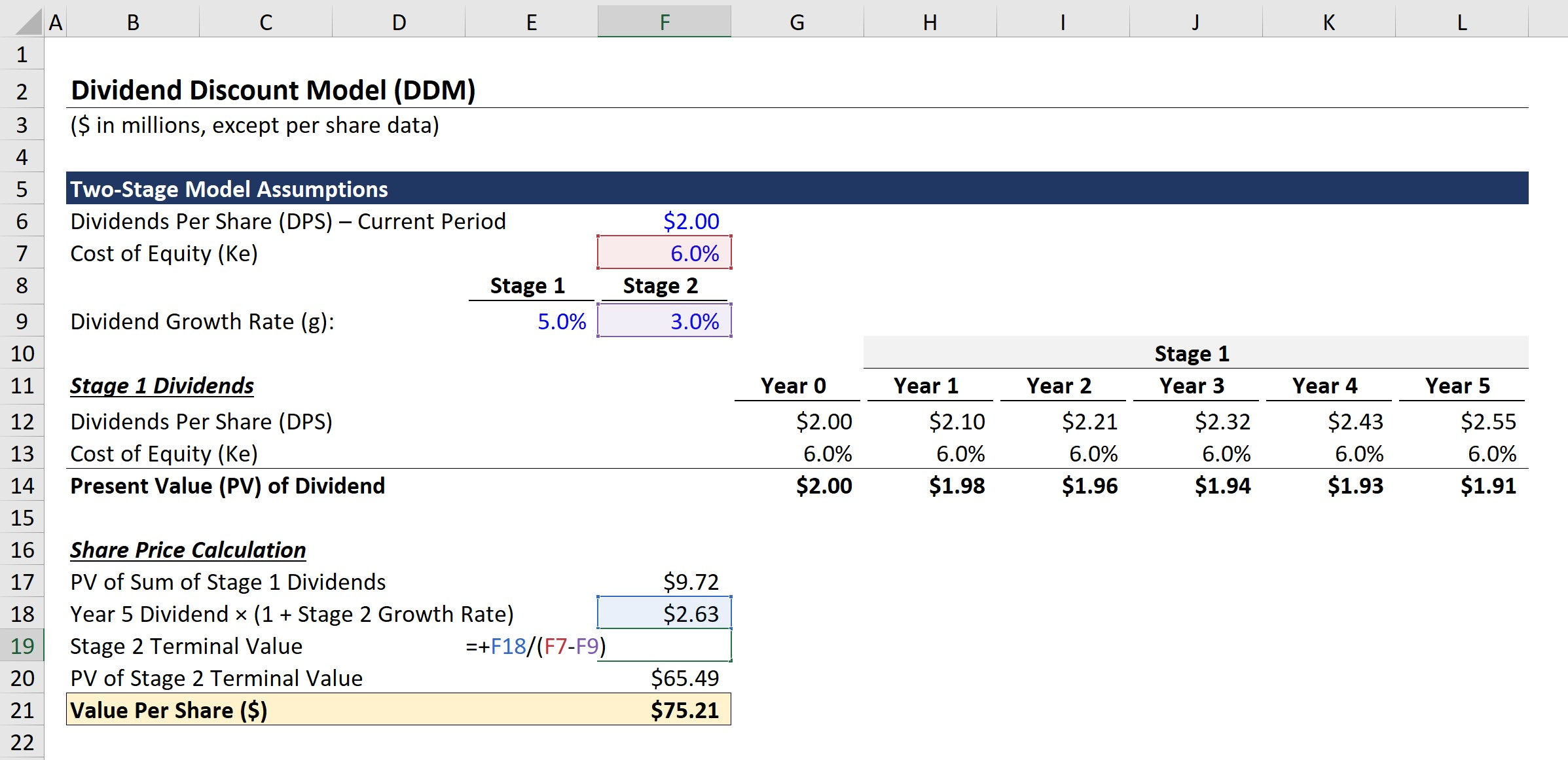But since the valuation is based on the present date, we must discount the terminal value by dividing \$87.64 by (1 + 6%)^5.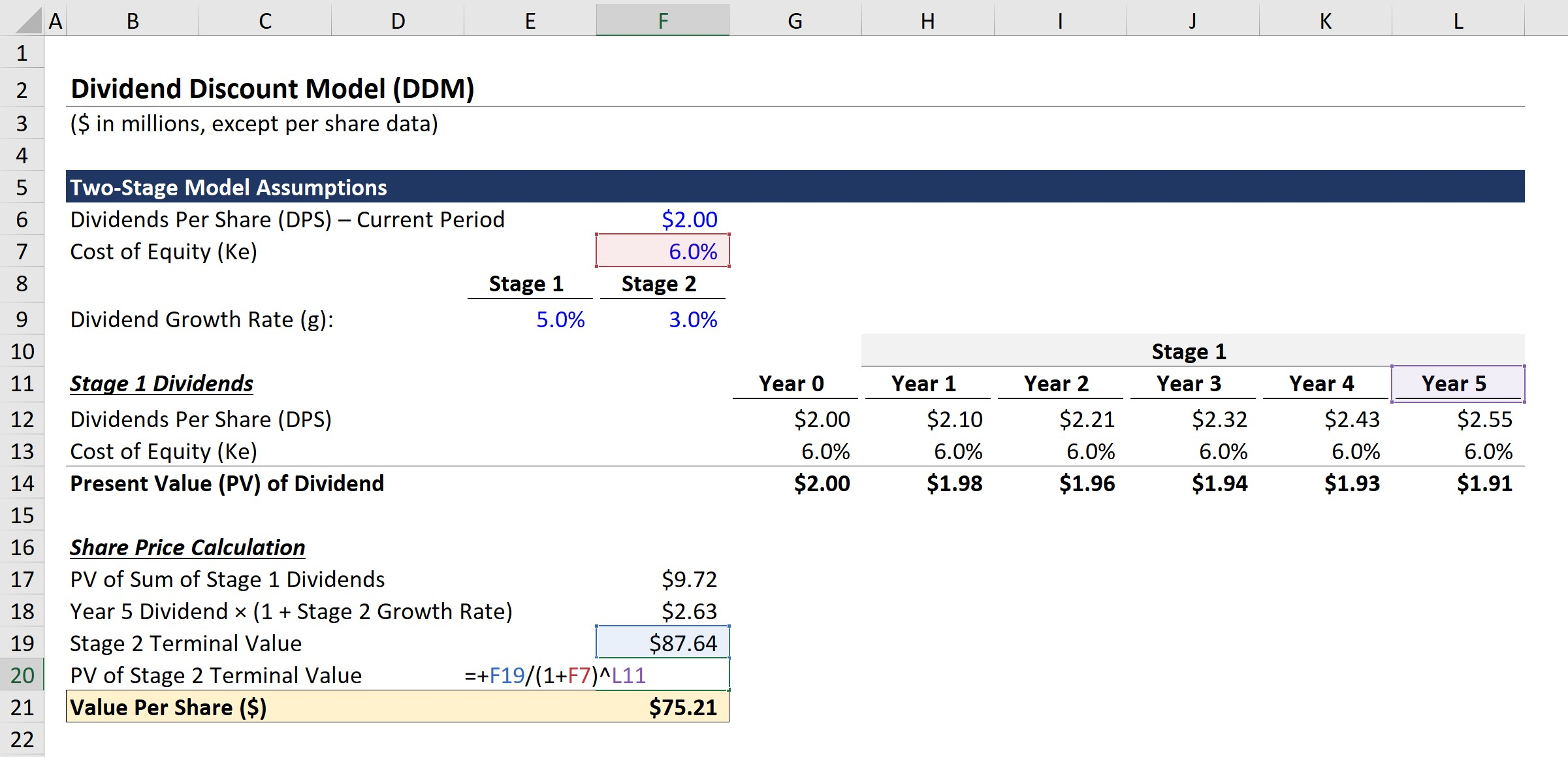## 3. Two-Stage DDM Implied Share Price Analysis

In the final step, the PV of the Stage 1 phase is added to the PV of the Stage 2 terminal value.

• Value Per Share (\$) = \$9.72 + \$65.49 = \$75.21

In conclusion, the implied share price derived from our two-stage dividend discount model (DDM) is \$75.21.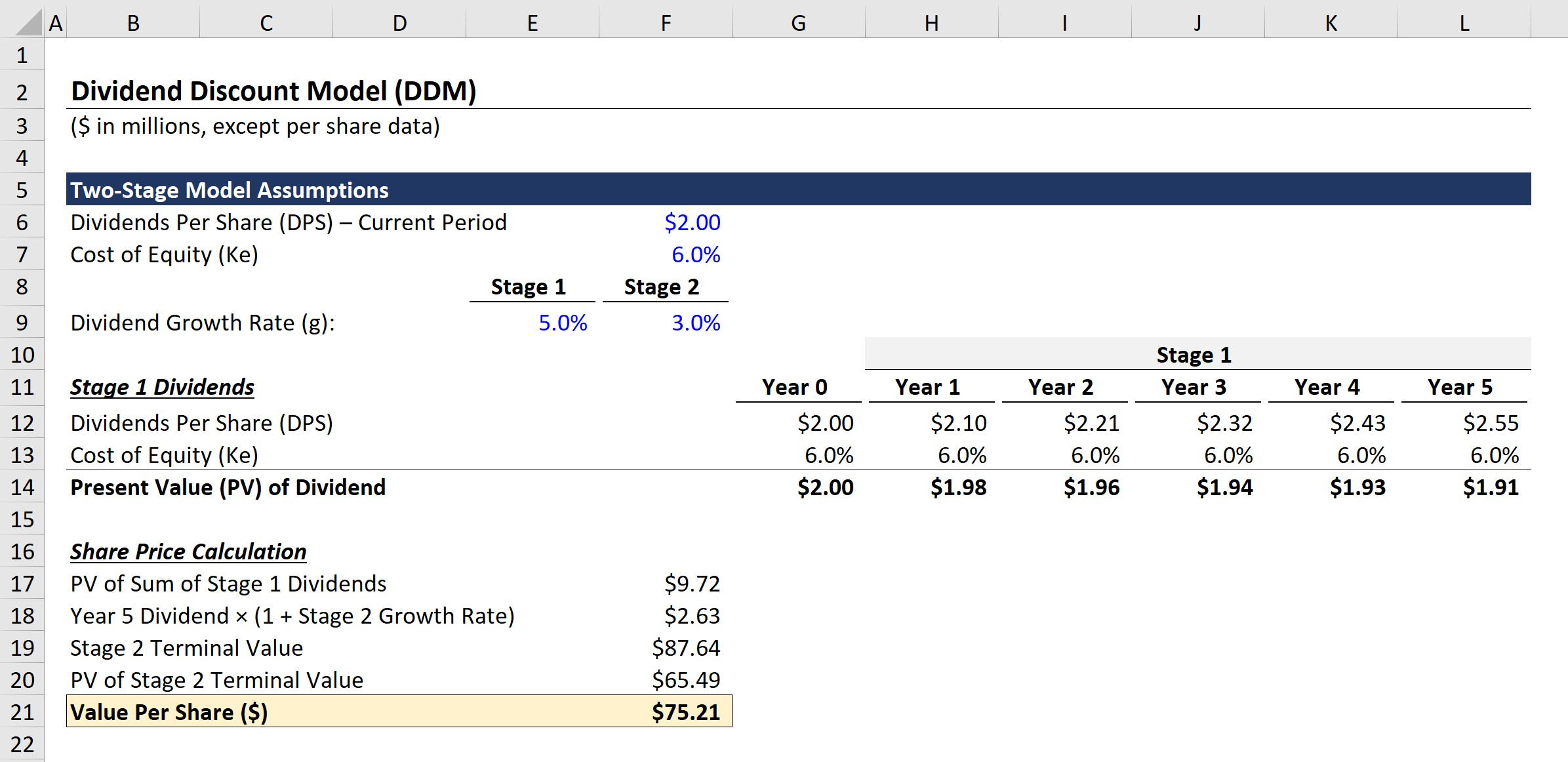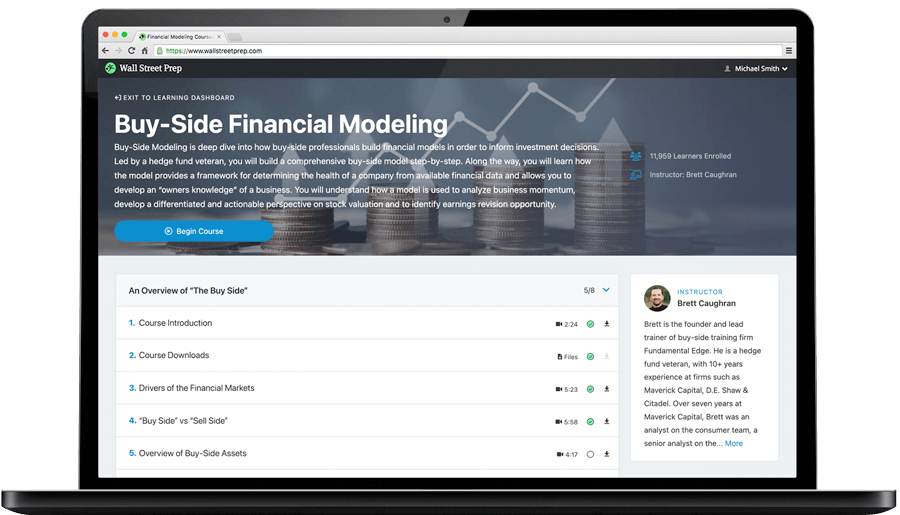Step-by-Step Online Course

### Learn Buy-Side (Hedge Fund) Modeling

Led by a former hedge fund PM (Maverick, Citadel, DE Shaw, Schonfeld), this program begins where financial modeling training ends — with a deep-dive into how buy-side analysts build financial models to make key investment decisions.

Inline Feedbacks